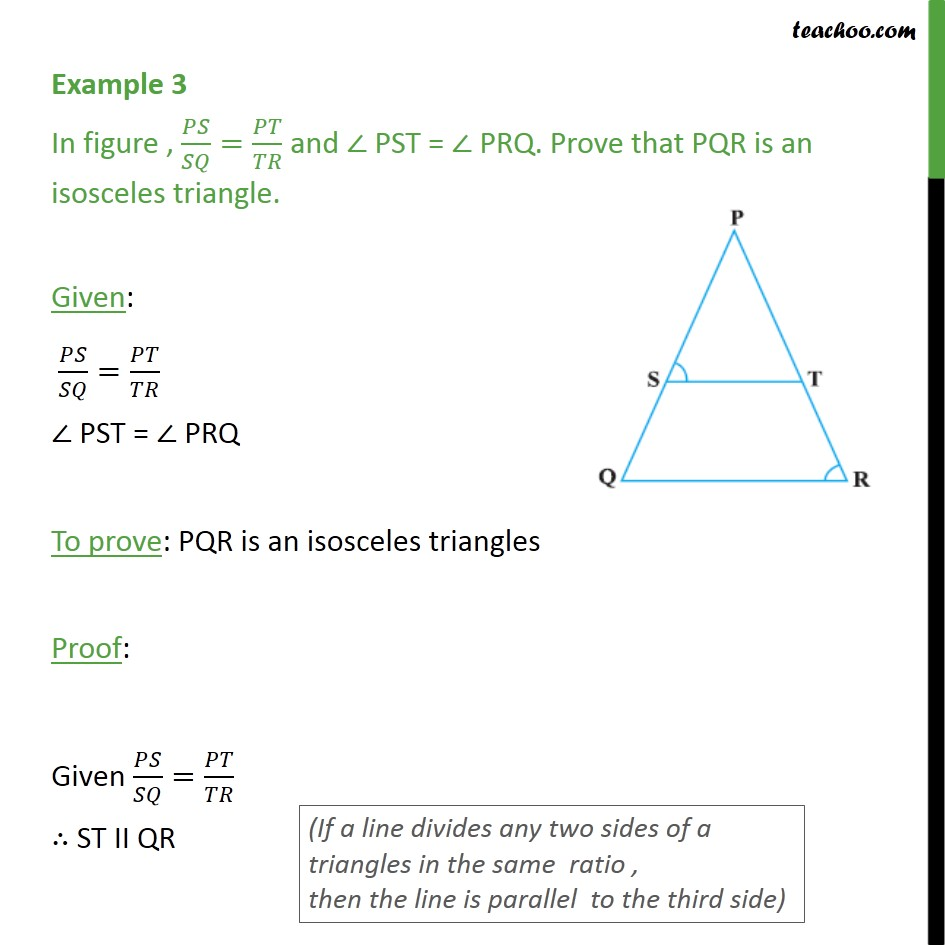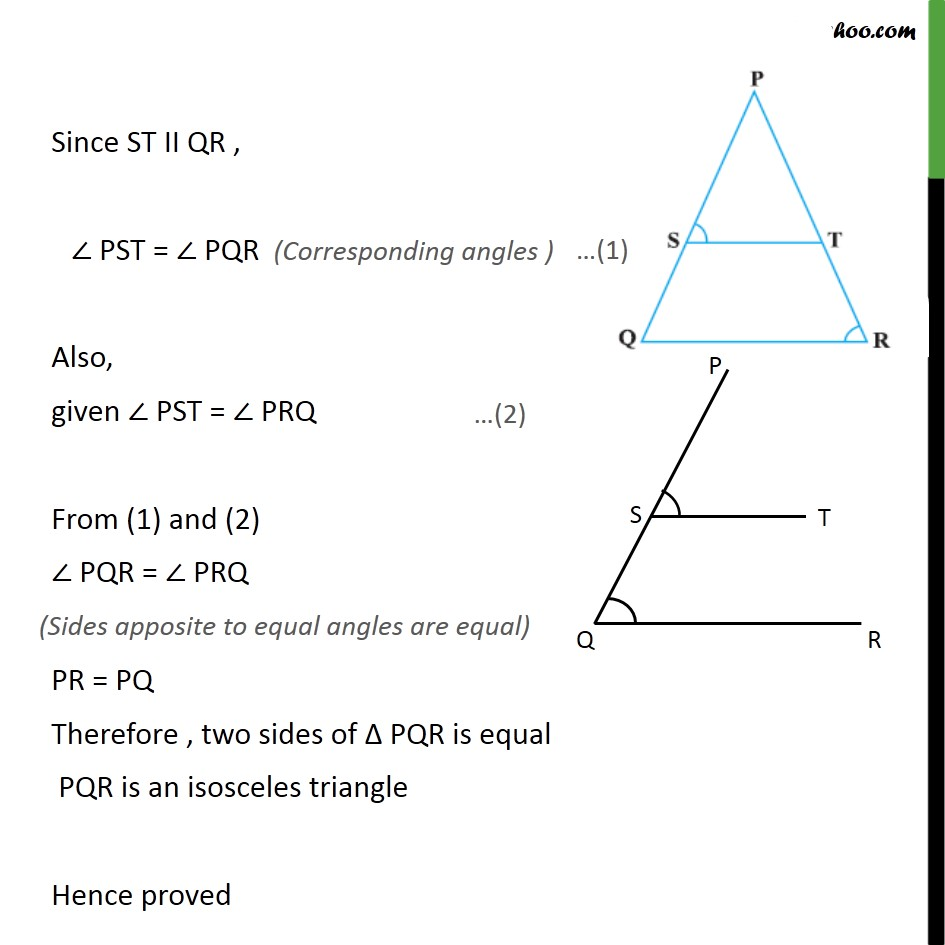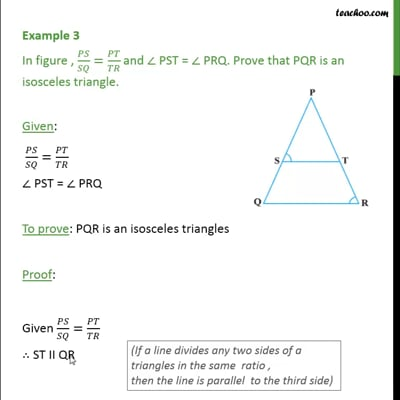Examples

Chapter 6 Class 10 Triangles (Term 1)
Serial order wiseThis video is only available for Teachoo black users

### Transcript

Example 3 In figure , / = / and PST = PRQ. Prove that PQR is an isosceles triangle. Given: / = / PST = PRQ To prove: PQR is an isosceles triangles Proof: Given / = / ST II QR Since ST II QR , PST = PQR Also, given PST = PRQ From (1) and (2) PQR = PRQ PR = PQ Therefore , two sides of PQR is equal PQR is an isosceles triangle Hence proved